Study Guide

## Surface Area

Nets aren't that hard. By now, you've probably got them. Or maybe they've got you. Either way, it gets a little tedious to have to draw them over and over again. There must be a better way to find these surface areas. And there is!

• ### Lateral Area of Prisms and Cylinders

Rather than busting open 3D solids like they're set pieces in a Hollywood movie, we can use lateral faces and lateral edges to find the surface area.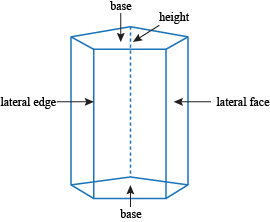A lateral face is any face that's not a base. But while a base is still a face, a lateral face is not a base. Is this the place for our rhyming grace? We don't think that this is the case. A lateral edge is the intersection of two lateral faces. Sounds either germy or painful.

The altitude of the prism is its height. Well why don't they just say that, then?

Sometimes, the lateral edge of a prism and the altitude are the same. That's called a right prism because the lateral edge makes a right angle with the base. An oblique prism doesn't do that, which is just not right.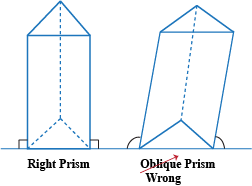The lateral area is the surface area of only the lateral faces. The lateral area L of all prisms makes a big rectangle. The lateral area of a right prism will be a rectangle with an altitude of height h and a width equal to the perimeter P of the base. It doesn't matter if the base looks like this: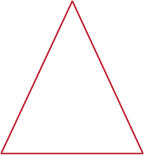Or this: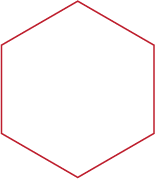Or even this:### Sample Problem

What is the lateral surface area of a regular heptagonal prism with a height of 15 feet and a base edge of 15 feet?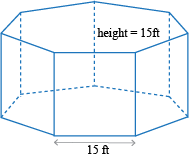Since the lateral area L equals the height h times the perimeter P of the base, we have the following.

L = Ph
L = (7 × 15 ft)(15 ft)
L = 1575 ft2

This same rule applies to cylinders, too. Cylinders are just like prisms except the base is a circle instead of a polygon.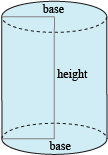The perimeter of a circle is actually a knight named Sir Cumference.

L = Ph
L
= (Circumference)h

Sir Cumference was a fairly round fellow who really liked pie. In fact, he liked it so much that he ordered two but ate the e's, so now he has 2π.

A circle's circumference equals 2π times the radius. So replace the perimeter with that, and we have the formula for the lateral area of a cylinder.

L = 2πrh

### Sample Problem

The Catfish Factory sells tuna, but is run by cats (because honestly, who else would work in that stink?). They need to figure out how big to make their labels that go around the cans of tuna if the cans are 2 inches tall and have diameters of 8 inches.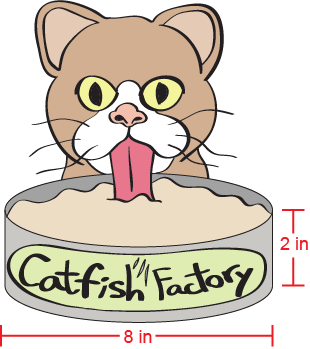The labels will go around the entire lateral area of the can. We'll start with the lateral area formula for cylinders:

L = 2πrh

The height of the can is 2 inches and the radius, which is half the diameter, is 4 inches.

L = 2π(4 in)(2 in)
L = 16π in2
L ≈ 50.3 in2

Now that they know how big to make their labels, the cats can sell (or lick) their cans of tuna in peace. It doesn't really matter since no one's going to buy canned tuna made by cats anyway. Can you say hairball?

• ### Surface Area of Prisms and Cylinders

We've calculated the lateral area for both prisms and cylinders. Lateral area is part of surface area, but it doesn't tell the whole story. We need the bases, too.No, not those bases.

The surface area SA equals the lateral area L plus the area of the two bases. Since the bases are congruent, they're identical. In Mathspeak,

SA = L + 2B

The ways to calculate L and B for cylinders and prisms are as different as night and later that night. We'll go through it and you'll see what we mean...hopefully.

### Sample Problem

This is a trapezoidal prism. Sounds more like a disease than a shape ("I have the trapezoidal prism flu!"). What is the surface area of the prism?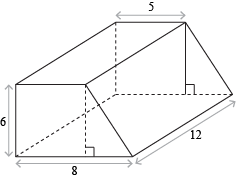We can find the surface area with all the formulas we know.

SA = L + 2B

We'll start with the lateral area, which is basically a big rectangle. We know that the area of a rectangle is bh and in this case, our b is really a P (the perimeter of the trapezoidal base). So our first step is to find P. Some say it's right before Q, but those might just be rumors.

We're missing one crucial side of P: the hypotenuse of the right triangle. Thankfully, we know the other two sides and the Pythagorean Theorem. Have at it.

a2 + b2 = c2
62 + (8 – 5)2 = c2
36 + 9 = c2
45 = c2
c ≈ 6.7

Hooray. Now we can find P, which is just the perimeter of the trapezoid.

P = 8 + 6 + 6.7 + 5
P = 25.7

We know our height, so we can find the lateral area.

L = Ph = (25.7)(12) = 308.4

We have part of the surface area. Now let's get back to the base-ics.

The trapezoid is just a rectangle and a triangle together. If we find the area of both and add them, we'll get the area of the base.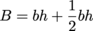The base of the rectangle is 5 and the base of the triangle is 3. The height of both is 6. Plug it in, plug it in.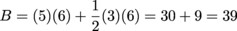Finally, we can find our surface area. Don't forget there are two bases in the mix.

SA = L + 2B
SA = 308.4 + 2(39) = 386.4 units2

Whew. We didn't even need chicken noodle soup beat the trapezoidal prism flu. We got through it all on our own.

What about the surface area of cylinders? If you ever want to gift-wrap some toilet paper (after the apocalypse, when rainforests are gone and paper is more expensive than diamonds), you'll need to know this one. Again, we'll start with the generic surface area formula.

SA = L + 2B

We know the lateral surface area is a rectangle with a height equal to the altitude and a width equal to the circumference.

L = 2πrh

Also, the bases are circles, with areas equal to πr2. Putting that all together, we have:

SA = 2πrh + 2(πr2)

### Sample Problem

What is the surface area of this cylinder?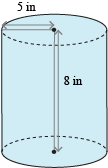We know the height and the radius, so we can just substitute them into the formula.

SA = 2π(5 in)(8 in) + 2π(5 in)2
SA = 80π + 50π = 130π = 408.4 in2

Congratulations. Now you can give your friends the gift of hygiene in a post-apocalyptic world. Of course, buying the wrapping paper might be just as expensive as buying the toilet paper itself.

• ### Surface Area of Pyramids

No offense to prisms and cylinders. They're great. But we don't eat our ice cream out of prisms while touring the Egyptian Cylinders of Giza. What we're saying is that there's no substitute for pyramids and cones, so we might as well learn a little bit about them.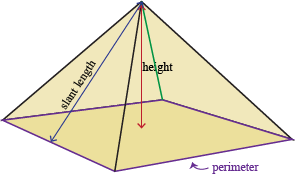A pyramid is a regular pyramid if the base is a regular polygon with a vertex that's perpendicular to the center of the base. A pyramid is irregular if it doesn't have daily bowel movements.

The height of the overall pyramid is called the altitude, but the lateral faces have their own height called the slant height. Let's take a regular hexagonal pyramid and look at its net (which looks surprisingly like the Star of David. L'chaim!).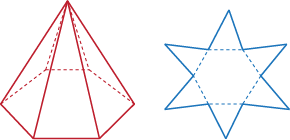The lateral area is just the area of all the triangles combined. The area of a triangle is half the base times the height. In this case, the base is the side of the hexagon s and the height of the triangle is the slant height l. Since we have six of these triangles, the lateral area is given by this equation: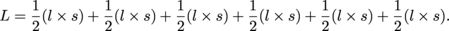Or, simplified, it equals: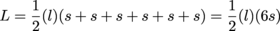Since we know the perimeter of the hexagon equals 6s, we can replace the sides with the perimeter of the hexagon P.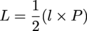Mazel tov! We've found the lateral area formula for any pyramid.

All that's missing for us to find the surface area is the base of the pyramid, B. Remember that unlike cylinders and prisms, pyramids only have one base. If we know the area of the base, we can find out the surface area of the whole pyramid.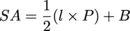### Sample Problem

For Father's Day, you decide to build your dad a birdhouse. You know how much he loves birds, and you'd rather do that than be his golf caddy for the millionth time. (Seriously, how many times can he call you "champ" in one day?) You decide that roof of the birdhouse will be a square pyramid because it's the easiest. The slant height of the roof is 10 inches and each side of the square is 8 inches. How much wood in square inches will you need to make the roof?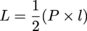The perimeter of the square base is 8 + 8 + 8 + 8 = 32 inches. The slant height that you want for the roof is 10 inches.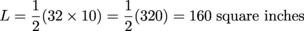Just be careful with that hacksaw. You don't want to end up like that woodshop teacher with the missing pinky.

We can use far more than these formulas to figure out surface area. Remember the Pythagorean Theorem? Remember trigonometry? Those mathematical ghosts will never leave you. As long as we treat them right, they'll be more like Casper and less like the Exorcist.

### Sample Problem

What is the surface area of this pentagonal pyramid?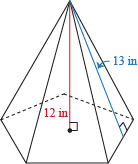Knowing the altitude and the slant height can give us enough information to calculate everything we need. If we look at those two values as a right triangle, the altitude is a leg and the slant height is the hypotenuse. Solving for the remaining leg (and hopefully it isn't prosthetic) will give us the distance from the center of the base to one of the sides.

a2 + b2 = c2
a2 + 122 = 132
a2 = 169 – 144
a2 = 25
a = 5

Quick tangent: the distance from the center of any regular polygon to the midpoint of one of its edges is called the apothem, which is what we just found.

Okay, tangent over. Now zoom in on the base of our pyramid. A perpendicular line segment from the center to the side is 5 inches (that's the apothem). Since the pentagon is regular, we can also find the angles in the right triangle. The 360° around the center is split into 5 evenly, which gives us 72° per isosceles triangle. We're splitting these triangles in half, so each angle is 36°. Here's what we know, Pictionary style.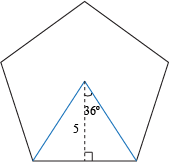Hey, speaking of going off on a tangent...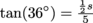We can solve for s and find the length of each side of the pentagon.

s = 10tan(36°) ≈ 7.27 inches

Be sure to thank trigonometry in your Nobel Prize acceptance speech.

Now we can do two things:

1. Find the area of the pentagonal base

2. Find the perimeter, which will help us find the lateral area

If you imagine cutting the pentagon into 5 identical triangles (feel free to imagine cutting it a little more if you're really mad at it), we can find the area of each triangle and multiply it by 5. The base of the triangle is the side length and the height is 5.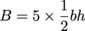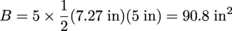Halfway done. If this were a romantic comedy, we'd be at the point where the two main characters have fallen in love already but don't know it yet, and some random conflict has just happened that tears them apart. That leaves just enough time for the epiphany of their love, the frantic last-minute chase for each other, and the obligatory saliva swap at the end.

The base is a pentagon ("penta-" means five), so perimeter is five times the length of the side. We know the side is about 7.27 inches, which means the perimeter is about 36.35 inches. High penta!

Now for the part where the two main characters realize their undying love and race to find each other. The lateral area of a pyramid is given by the formula:We know the slant height l is 13 since it was given to us, and we just calculated P.

L = ½(36.35 × 13) ≈ 236.2 in2

The two lovers have reunited and professed their love at long last. All that remains is that slobbery kiss that lasts for centuries.

SA = L + B
SA = 236.2 in2 + 90.8 in2 = 327 in2

The end. Roll credits to some feel-good Michael Bolton song.

• ### Surface Area of Cones

We've covered pyramids (literally, since we found the surface area), and now it's time to cover cones (literally, since we'll find the surface area).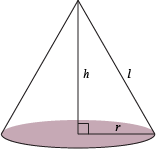A right cone is a cone where the axis is also the altitude. That means the height from the point on top to the base on the bottom hits the circle dead center at a 90° angle. All other cones are wrong. They're just plain wrong.

If we take a look at this cone's net, we'll be able to say something about its lateral and surface areas other than, "It's right here." Duh.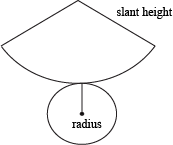The lateral area of the cone is really a sector of a circle with radius l. (It used to be the slant height, now it's the radius?) The arc length of the sector is the same as the circumference of the base circle.

Proportions have served us well in the past, and they'll continue to do that if we use them right. The lateral area is the area of the sector. If we compare that to the area of what would be the whole circle, we can compare the arc length to what would have been the circumference.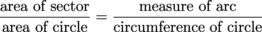The area of the sector is what we're trying to find. The area of the circle with radius l is πl2. The measure of the arc is the circumference of the smaller circle, 2πr, and the circumference of the bigger circle is 2πl.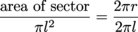You could go through rearranging and solving yourself, or just trust us that it'll look like this in the end:

Area of sector = πrl

That means the lateral area of a cone is equal to πrl. Unexpectedly simple. To get the total surface area of a cone, we add in the area of the base:

SA = πrl + πr2

### Sample Problem

The "Bigger Is Better" Ice Cream Company makes its own conical waffle cones. Their Super Duper Ice Cream Scooper is a scoop of ice cream that's 6 inches in diameter in a waffle cone. Mmmm. The cone itself has an altitude of 10 inches. How much waffle do they need to make the cone (in square inches)? And where's the closest store?

The diameter of the scoop is the diameter of the circular base of the cone. We're interested in the radius, not the diameter. (Hopefully he'll have better luck on match.com.) That means our radius r is 3 inches.

What about l, the slant height? The radius and the altitude form two legs of a right triangle with the slant height as the hypotenuse. Pythagorize it up.

a2 + b2 = c2
32 + 102 = c2
109 = c2
c ≈ 10.44 inches

Now that we've found our slant height, we can find the waffle area using the lateral area formula for a cone.

L = πrl
L = π(3 inches)(10.44 inches)
L ≈ 98.4 square inches

Bigger really is better.

Like a pyramid, the surface area of an entire cone (base included), is just the lateral area plus the area of the base.

SA = L + B

We know the lateral area of a cone is πrl. The base of the cone is a circle with area πr2. Plug those in, and we've got a surface area formula.

SA = πrl + πr2

• ### Surface Area of Spheres

If you play basketball, eat oranges, or have eyeballs (which are sort of necessary to read this), you've most likely encountered spheres at some point in your life. They're common everyday objects, so learning how to find their surface area should prove useful...you know, in case you've ever wondered how much peel is actually on an orange.

Before we do that though, we need to talk about the Great Circle of Life.

Er...sort of. A great circle is a circle that has the same center and radius as its sphere. Imagine cutting a sphere exactly in half, creating two hemispheres. The circle we get is a great circle. Circles anywhere else on the sphere aren't that great. They're mediocre.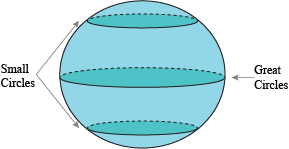How does this help us find the surface area? Patience, young grasshopper.

The great circle (with area πr2, obv) covers about a fourth of the sphere. Actually, it covers exactly a fourth of the sphere. That means our surface area for the entire sphere is four times the area of the great circle.

SA = 4πr2

Coolness. We can get started on those surface areas. Hold your applause, please.

### Sample Problem

What is the surface area of this sphere?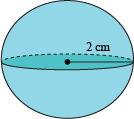SA = 4πr2

Our radius is 2 centimeters. That's all we need.

SA = 4π(2 cm)2
SA = 16π ≈ 50.3 cm2

Could it get any simpler? Probably not. Could it get any harder? Unfortunately, always.

### Sample Problem

If we slice the following sphere into two identical hemispheres, what's the surface area of one hemisphere?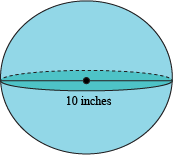This time, our formula is going to be a smidgeon more complicated. We're looking for the surface area of the hemisphere, which is half the surface area of the sphere plus the area of the great circle.

SA = 2πr2 + πr2 = 3πr2

Our diameter is 10 inches, so our radius is 5 inches.

SA = 3πr2
SA = 3π(5 in)2
SA = 75π ≈ 235.6 in2

That's all there is to it. Well, let's throw in one more curveball to see if you really get it. Get it? Because a curveball is a sphere, too!

### Sample Problem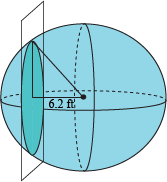The small circle intersects the sphere where its center is 6.2 feet away from the center of the sphere. If the radius of the small circle is 5 feet, what is the surface area of the sphere?

Whoa, whoa, whoa. What in the name of Ke\$ha is going on here? Let's make sense out of all of this.

The distance between the center of the circle and the sphere is 6.2 feet, which makes a right angle with the \$5 footlong radius of the circle. If we make it a right triangle and look at the hypotenuse, it's the light at the end of this spherical tunnel: the radius of the sphere.

The Pythagorean Theorem, like the Energizer bunny, keeps going on and on and on…

a2 + b2 = c2

Substitute in the values we know.

(6.2 ft)2 + (5 ft)2 = c2

And solve for the sphere's radius.

c = r ≈ 7.96 ft

Once we have that, surface area's a breeze.

SA = 4πr2
SA = 4π(7.96 ft)2
SA ≈ 796 ft2